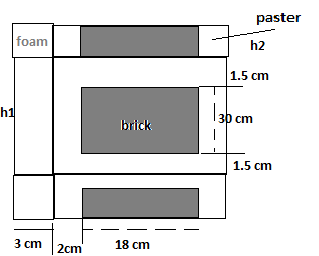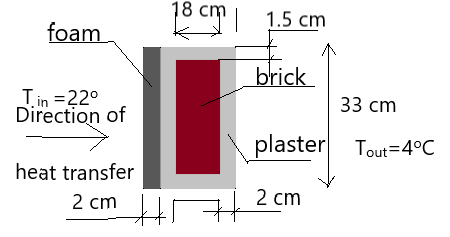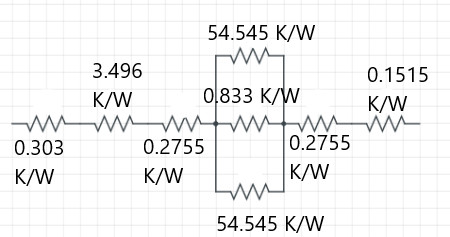# A 4 m high and 6 m wide wall consists of a long 18 cm by 30 cm cross section of horizontal bricks...

## Question:

A 4 m high and 6 m wide wall consists of a long 18 cm by 30 cm cross section of horizontal bricks ( {eq}k = 0.72 \space W/m \space ^\circ C {/eq}) separated by 3 cm thick plaster layers {eq}(k = 0.22 \space W/m \space ^\circ C) {/eq}. There are also 2 cm thick plaster layers on each side of the wall and a 3 cm thick rigid foam {eq}(k = 0.026 \space W/m \space ^\circ C) {/eq} on the inner side of the wall. The indoor and outdoor temperatures are {eq}22^\circ C {/eq} and {eq}4^\circ C {/eq} and convention heat transfer coefficients on the inner and outer sides are {eq}h_1 = 10 \space W/m^2 \space ^\circ C,h_2 = 20 \space W/m^2 \space ^\circ C {/eq} respectively. Assuming one dimensional heat transfer and disregarding radiation, determine the rate of heat transfer through the wall.## Heat transfer with combined convection and conduction:

Heat transfer by conduction is predominant in solids. Heat transfer by convection is predominant in fluids.There are cases when both conduction and convection resistance are there simultaneously and then we use electrical analogy to get the equivalent resistance offered to the heat flow.

The following figure shows the cross-section of the wall as follows;From Fourier's law of heat conduction for 1-dimensional steady heat transfer through a wall, we have

{eq}\begin{align*} Q &= kA \frac { \Delta T } { \Delta x } \\ &= \frac { \Delta T } { \frac { \Delta x } { kA } } \\ &= \frac { \Delta T } { \Sigma R_{th} } \\ \end{align*} {/eq}

where

{eq}\begin{align*} Q &= \text { Heat transfer through the wall } \\ k &= \text { thermal conductivity of the material of the wall } \\ A &= \text { cross sectional area of the wall perpendicular to the heat transfer direction } \\ \Delta T &= \text {Temperature difference across the wall } \\ \Delta x &= \text {Thickness of the wall } \\ \Sigma R_{th} &= \text { Total resistance offered to the heat flow } \\ \end{align*} {/eq}

We can compare this formula {eq}Q = \frac { \Delta T } { \Sigma R_{th} } {/eq} with Ohm's law {eq}I = \frac { V } { R } {/eq}.Just like voltage is the driver of current and electrical resistance hinders the flow of current, similarly , temperature difference is the driver of heat transfer and thermal resistances hinder the flow of heat . So, the way we combine electrical resistances can be applied to thermal resistances as well.

Thus, using the electrical resistance analogy, we have the following thermal resistance diagram for the given problem ;Calculation of the various thermal resistances shown in the figure;

First of all, the brick and plaster repeats itself over and over to complete the wall. A height of 33 cm in the vertical direction includes brick plaster system that can be considered as a unit that repeats itself over and over to complete the wall. In the horizontal direction, the wall is uniform, so we take a depth (into the plane of the screen ) of 1 m.

So, we consider an area of 0.33 m^2 for our analysis.

{eq}\begin{align*} A_1 &= 0.33 \times 1 \\ &= 0.33 \ m^2 \\ \end{align*} {/eq}

Starting from the inside of the wall, the first thermal resistance we encounter is convection resistance. It is calculated as follows;

{eq}\begin{align*} R_1 &= \frac { 1 } { h_1 A_1 } \\ \end{align*} {/eq}

Given

{eq}h_1 = 10 \space W/m^2 \space ^\circ C \\ {/eq}

We have

{eq}\begin{align*} R_1 &= \frac { 1 } { h_1 A_1 } \\ &= \frac { 1 } { 10 \times 0.33 } \\ &= 0.303 \ K/W \\ \end{align*} {/eq}

Next resistance is the conduction resistance offered by foam.

Given:

{eq}\begin{align*} k_{f} &= 0.026 \ W/mK \\ A_{f} &= A_1 \\ &= 0.33 \ m^2 \\ \Delta x_{f} &= 0.03 \ m \\ \end{align*} {/eq}

Thus,

{eq}\begin{align*} R_2 &= \frac { \Delta x_{f} } { k_f A_f } \\ &= \frac { 0.03 } { 0.026 \times 0.33 } \\ &= 3.496 \ K/W \\ \end{align*} {/eq}

Next resistance is the conduction resistance offered by plaster.

Given:

{eq}\begin{align*} k_{P} &= 0.22 \ W/mK \\ A_{P} &= A_1 \\ &= 0.33 \ m^2 \\ \Delta x_{P} &= 0.02 \ m \\ \end{align*} {/eq}

Thus,

{eq}\begin{align*} R_3 &= \frac { \Delta x_{P} } { k_P A_P } \\ &= \frac { 0.02 } { 0.22 \times 0.33 } \\ &= 0.2755 \ K/W \\ \end{align*} {/eq}

Till now the resistances were in series. Now we have three resistances in parallel.

The first one is plaster and its different attributes are as follows;

Given:

{eq}\begin{align*} k_{p} &= 0.22 \ W/mK \\ A_{p} &= A_2 \\ &=0.015 \times 1 \\ &= 0.015 \ m^2 \\ \Delta x_{p} &= 0.18 \ m \\ \end{align*} {/eq}

Thus,

{eq}\begin{align*} R_4 &= \frac { \Delta x_{p} } { k_p A_p } \\ &= \frac { 0.18 } { 0.22 \times 0.015 } \\ &= 54.545 K/W \\ \end{align*} {/eq}

The next resistance is the brick with the following attributes;

{eq}\begin{align*} k_{b} &= 0.72 \ W/mK \\ A_{b} &= A_3 \\ &=0.3 \times 1 \\ &= 0.3\ m^2 \\ \Delta x_{b} &= 0.18 \ m \\ \end{align*} {/eq}

{eq}\begin{align*} R_5 &= \frac { \Delta x_{b} } { k_b A_b } \\ &= \frac { 0.18 } { 0.72 \times 0.3 } \\ &= 0.833 \ K/W \\ \end{align*} {/eq}

The next resistance is again plaster and it is similar to {eq}R_4 {/eq}.

Thus,

{eq}\begin{align*} R_6 &= R_4 \\ &= 54.545 \ K/W \\ \end{align*} {/eq}

The next resistance is again plaster and it is similar to {eq}R_3 {/eq}.

Thus,

{eq}\begin{align*} R_7 &= R_3 \\ &= 0.2755 \ K/W \\ \end{align*} {/eq}

The last resistance is convection resistance of air outside and is calculated as follows;

{eq}\begin{align*} R_8 &= \frac { 1 } { h_2 A_1 } \\ &= \frac { 1 } { 20 \times 0.33 } \\ &= 0.1515 \ K/W \\ \end{align*} {/eq}

This gives the resistance network as follows;First, solve the thermal resistances in parallel and then all resistances will come in series. The final equivalent resistance will be

{eq}\begin{align*} \Sigma R_{th} &= 5.3098 \ K/W \\ \end{align*} {/eq}

So, heat transfer from an area of 0.33 m^2 is calculated as follows;

{eq}\begin{align*} q &= \frac {\Delta T } { \Sigma R_{th} } \\ &= \frac { (22 - 4) ^o \ C } { 5.3098 } \\ &= 3.389 \ W \\ \end{align*} {/eq}

Now, heat transfer from the whole of the wall is calculated as follows ;

{eq}\begin{align*} Q &= { 3.389 } { 0.33 } \times (\text {Area of wall }) \\ &= \frac { 3.389 } { 0.33 } \times (6 \times 4) \\ &= 246.472 \ W \\ \end{align*} {/eq}